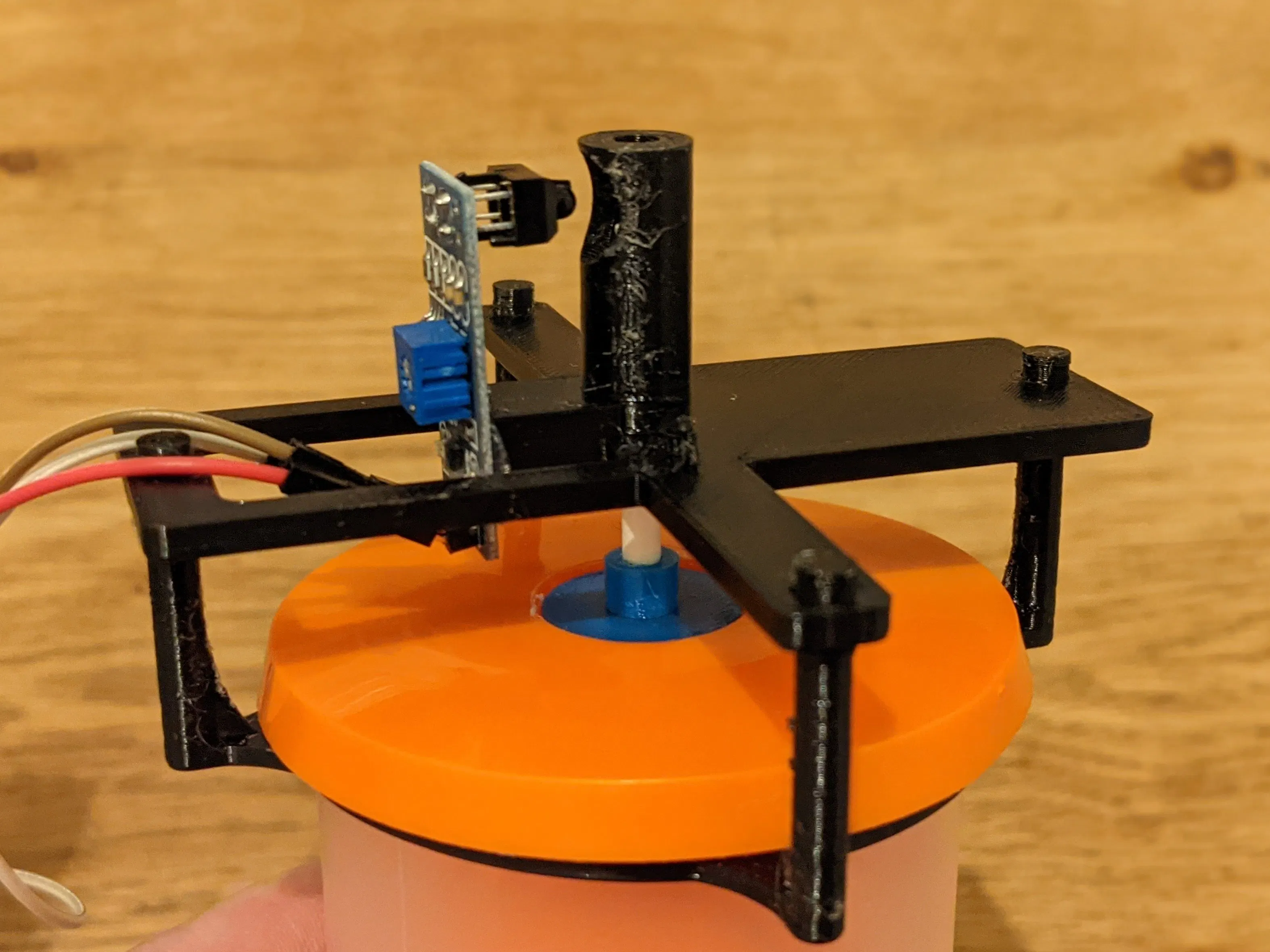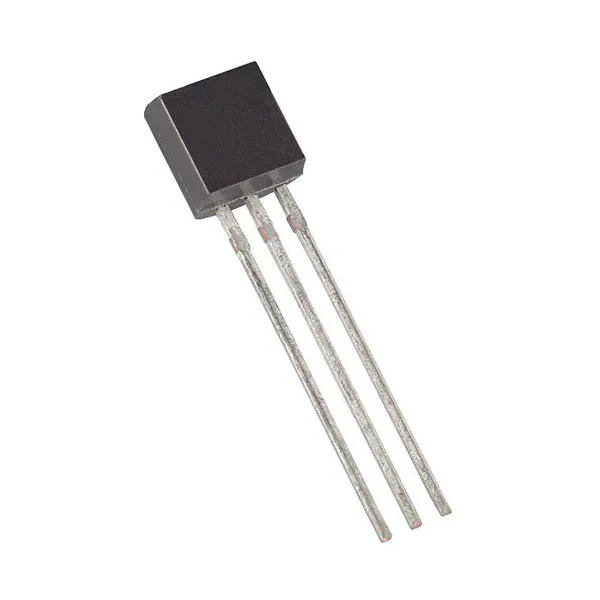# DIY Fermentation Blubb Counter for Home Brewing

Keep track of fermentation status via this LoRaWAN-powered low-cost fermentation counter. Track the fermentation "blubbs" and temperature.

IntermediateFull instructions provided5 hours3,788## Things used in this project

### Hardware components

 Heltec CubeCell HTCC-AB01
×1
 Line-Following Sensor
×1Maxim Integrated DS18B20 Programmable Resolution 1-Wire Digital Thermometer
×1

### Software apps and online servicesArduino IDE

### Hand tools and fabrication machines3D Printer (generic)
 Cake Pop Sticks, 4mm

## Custom parts and enclosures

### Ring

Attaches to the Speidel Fermentation Lock

### SensorHolder

Attached to the ring STL and holds sensors and CubeCell board.

### Stick Attachment

Holds the cake pop stick

## Code

### CubeCell LoraWAN for TTN with Fermentation Sensor Code

Arduino
Make sure you created a TTN application and device - add your own network security and app / device IDs.
```#include <OneWire.h>
#include <DallasTemperature.h>
#include "LoRaWan_APP.h"
#include "Arduino.h"

// Data wire is plugged into GPIO5 on the CubeCell
#define ONE_WIRE_BUS GPIO5

// Setup a oneWire instance to communicate with any OneWire devices (not just Maxim/Dallas temperature ICs)
OneWire oneWire(ONE_WIRE_BUS);

// Pass our oneWire reference to Dallas Temperature.
DallasTemperature sensors(&oneWire);

/*
set LoraWan_RGB to Active,the RGB active in loraWan
RGB red means sending;
RGB purple means joined done;
RGB blue means RxWindow1;
RGB yellow means RxWindow2;
*/

int threshold = 0;
static unsigned int counter = 0;
static byte interval = 0;
boolean lastState = false;
unsigned long lastSend;
unsigned long sendInterval = 600000; //every 10 mins
unsigned long lastBlubb;

/* OTAA para USED*/
uint8_t devEui[] = { xxx };
uint8_t appEui[] = { xxx };
uint8_t appKey[] = { xxx };

/* ABP para NOT USED*/
uint8_t nwkSKey[] = { xxx };
uint8_t appSKey[] = { xxx };
uint32_t devAddr =  ( uint32_t )0x007e6ae1;

uint16_t userChannelsMask = { 0x00FF, 0x0000, 0x0000, 0x0000, 0x0000, 0x0000 };

/*LoraWan region, select in arduino IDE tools*/
LoRaMacRegion_t loraWanRegion = ACTIVE_REGION;

/*LoraWan Class, Class A and Class C are supported*/
DeviceClass_t  loraWanClass = LORAWAN_CLASS;

/*the application data transmission duty cycle.  value in [ms].*/
uint32_t appTxDutyCycle = 500;

/*OTAA or ABP*/
bool overTheAirActivation = LORAWAN_NETMODE;

/* set LORAWAN_Net_Reserve ON, the node could save the network info to flash, when node reset not need to join again */
bool keepNet = LORAWAN_NET_RESERVE;

/* Indicates if the node is sending confirmed or unconfirmed messages */

/* Application port */
uint8_t appPort = 2;
/*!
Number of trials to transmit the frame, if the LoRaMAC layer did not
according to the LoRaWAN Specification V1.0.2, chapter 18.4, according
to the following table:

Transmission nb | Data Rate
----------------|-----------
1 (first)       | DR
2               | DR
3               | max(DR-1,0)
4               | max(DR-1,0)
5               | max(DR-2,0)
6               | max(DR-2,0)
7               | max(DR-3,0)
8               | max(DR-3,0)

Note, that if NbTrials is set to 1 or 2, the MAC will not decrease
the datarate, in case the LoRaMAC layer did not receive an acknowledgment
*/
uint8_t confirmedNbTrials = 4; //was 4

/* Prepares the payload of the frame */
static void prepareTxFrame( uint8_t port )
{
/*appData size is LORAWAN_APP_DATA_MAX_SIZE which is defined in "commissioning.h".
appDataSize max value is LORAWAN_APP_DATA_MAX_SIZE.
if enabled AT, don't modify LORAWAN_APP_DATA_MAX_SIZE, it may cause system hanging or failure.
if disabled AT, LORAWAN_APP_DATA_MAX_SIZE can be modified, the max value is reference to lorawan region and SF.
for example, if use REGION_CN470,
the max value for different DR can be found in MaxPayloadOfDatarateCN470 refer to DataratesCN470 and BandwidthsCN470 in "RegionCN470.h".
*/
appDataSize = 4;

appData = (counter >> 8);
appData = counter;
appData = interval;
appData = getByteTemp();
}

void setup() {
boardInitMcu();
lastSend = millis();
lastBlubb = millis();
Serial.begin(115200);

//set threshold value (stick not visible)
int total = 0;
for (int i = 0; i < 5; i++) {
}

int average = total / 5;

int fivePercent = average / 100 * 5;

threshold = (total / 5) - fivePercent;

//init dallas temp sensor
sensors.begin();

#if(AT_SUPPORT)
enableAt();
#endif
deviceState = DEVICE_STATE_INIT;
LoRaWAN.ifskipjoin();
}

void loop()
{
blubb();

switch ( deviceState )
{
case DEVICE_STATE_INIT:
{
#if(AT_SUPPORT)
getDevParam();
#endif
printDevParam();
LoRaWAN.init(loraWanClass, loraWanRegion);
deviceState = DEVICE_STATE_JOIN;
break;
}
case DEVICE_STATE_JOIN:
{
LoRaWAN.join();
break;
}
case DEVICE_STATE_SEND:
{

if (millis() > (lastSend + sendInterval)) {
prepareTxFrame( appPort );
LoRaWAN.send();
lastSend = millis();
}
deviceState = DEVICE_STATE_CYCLE;
break;
}
case DEVICE_STATE_CYCLE:
{
// Schedule next packet transmission
txDutyCycleTime = appTxDutyCycle;// + randr( 0, APP_TX_DUTYCYCLE_RND );
LoRaWAN.cycle(txDutyCycleTime);
deviceState = DEVICE_STATE_SLEEP;
break;
}
case DEVICE_STATE_SLEEP:
{
LoRaWAN.sleep();
break;
}
default:
{
deviceState = DEVICE_STATE_INIT;
break;
}
}
}

void blubb() {
boolean state = getState();
//if the cake stick is in down position
if (lastState != state) {

if (state == false && (millis() + 1500) > lastBlubb)
{
counter = counter + 1;
signalBlubb(counter);

//this is just roughly ok, state=false has a 5% threshold
//also, as we are not transmitting on every blubb, there is a natural
//variation here. A chart trendline will be really useful.
//just seconds
float rawInterval = ((millis() - lastBlubb) / 1000);
if (rawInterval > 255) {
interval = 255;
} else {
interval = rawInterval; //reduce to byte
}

lastBlubb = millis();

lastState = state;
} else if (state == true) {
lastState = state;
}

}

delay(50);
}

void signalBlubb(unsigned int counter) {
Serial.print("blubb ");
Serial.println(counter);

turnOnRGB(getColor ( 255, 255, 255 ), 50 );
turnOffRGB();
}

uint32_t getColor(uint8_t r, uint8_t g, uint8_t b) {
return ((uint32_t)r << 16) | ((uint32_t)g <<  8) | b;
}

boolean getState() {
int total = 0;
int sensorValue = 0;
for (byte i = 0; i<10; i++)
{
delay(5);
}

sensorValue = total / 10;

Serial.print(sensorValue);
bool state = (sensorValue < threshold) ? true : false;
Serial.print(" ");
Serial.print(state ? "true" : "false");
Serial.print(" - threshold  ");
Serial.println(threshold);
return state;
}

byte getByteTemp() {
byte byteTemp = (getTemp() - 5) * 10;
Serial.print("Byte temp (div by 10 then plus 5 ) is:  ");
Serial.println(byteTemp);
return byteTemp;
}

float getTemp() {
sensors.requestTemperatures(); // Send the command to get temperatures
float tempC = sensors.getTempCByIndex(0);
Serial.print ("TempC is ");
Serial.print (tempC);
return tempC;

}
```

### OpenSCAD File for all 3d printed parts

```\$fn=128;

color("red", 0.75)
ring();

color("blue", 0.75)
translate([0,0,30])
sensorHolder();

color("green", 0.75)
translate([0,0,50])
cakeStick();

color("pink", 0.75)
translate([0,0,15])
cakeStickAttachment();

color("violet", 0.75)
translate([15.5,0,38.5])
rotate([0,-90,0])
sensor();

color("yellow", 0.75)
translate([-22,0,36])
rotate([0,0,90])
cubeCell();

module cubeCell() {
cube([41.5, 25, 7.6], center=true);
}

module sensor() {
difference() {
cube([32.5,19.5,1.5], center = true);

translate([-8.5,0,0])
cylinder(d=3.5, h=2, center=true);

}
translate([12.8,0,5.3])
cube([7, 11, 12], center = true);

translate([-13.5,0,1.4])
cube([3, 17, 2], center = true);

translate([1,3,1.4])
cube([8, 6, 2], center = true);

}

//4.2 diameter easy flow
//4.0 stick to filament
module cakeStickAttachment() {
cylinder(d=19, h=0.5, center=true);

translate([0,0,2.5])
difference() {
cylinder(d=7,h=5, center=true);
cylinder(d=3.8,h=5.1, center=true);
}
}

//15 x 0.4 x 0.4
module cakeStick() {
cylinder(d=4.0,h=50, center=true);
}

module sensorHolder() {
difference() {
union() {
handle();

//90deg rotated and spaced
spacedHandle();

translate([0,0,13])
difference() {
cylinder(d=17,h=28, center=true);
cylinder(d=4.3,h=28.1, center=true);
translate([6,0,8.5])
sphere(d=10);
}

translate([10,0,0.5])
difference() {
cube([15,2,3], center=true);
translate([5.5,0,1.1])
cube([10,2.1,1], center=true);
}

difference() {
translate([9,0,6])
cube([12,16,14], center=true);

translate([15.8,0,8.75])
rotate([0,-90,0])
sensor();

}

//cable holder 2x
translate([0,-12.5,4])
cableHolder();
translate([0,12.5,4])
cableHolder();

//antenna holder
translate([0,-34,4])
cableHolder();

//cable holder on the side for temp sensor
translate([46.5,15.5,0.5])
rotate([0,0,0])
difference() {
cylinder(d=10,h=3, center=true);
cylinder(d=5, h=3.1, center=true);
}
}

cylinder(d=4.3,h=4.1, center=true);

}

}

module cableHolder() {

rotate([0,90,0])
difference() {
cylinder(d=10,h=2, center=true);
cylinder(d=5, h=2.1, center=true);
}

}

module spacedHandle() {

rotate([0,0,90])
difference () {
minkowski() {
cube([25,86,2], center=true);
cylinder(r=2,h=1);
}

minkowski() {
cube([17,72.5,2.1], center=true);
cylinder(r=2,h=1);
}

translate([0,41.5,0])
cylinder(d=4.8,h=4.1, center=true);

translate([0,-41.5,0])
cylinder(d=4.8,h=4.1, center=true);
}

}

module handle() {
difference () {
minkowski() {
cube([5,86,2], center=true);
cylinder(r=2,h=1);
}

translate([0,41.5,0])
cylinder(d=4.8,h=4.1, center=true);

translate([0,-41.5,0])
cylinder(d=4.8,h=4.1, center=true);
}

}

module ring() {
difference() {
cylinder(h=2, r=33.25, center=true);
cylinder(h=2.1, r=30.50, center=true);
}

translate([0,-33.5,0])
stick();

translate([0,33.5,0])
rotate([0,0,180])
stick();

translate([33.5,0,0])
rotate([0,0,90])
stick();

translate([-33.5,0,0])
rotate([0,0,-90])
stick();

}

module stick() {
translate([0,-3,0])
cube([4.5,10,2], center=true);

translate([0,-8,14])
cylinder(h=30, r=2.25, center=true);

translate([-4.9,0,0])
rotate([0,0,180])
roundEdge();

translate([4.9,0,0])
rotate([0,180,180])
roundEdge();

translate([0,-4.5,5])
rotate([0,-90,0])
scale([1.5,0.5,0.5])
roundEdge();

translate([0,-8,23])
sphere(d=5);

}

module roundEdge() {
difference() {
cylinder(r=10, h=2, center=true);

translate([8,8,0])
cylinder(r=11, h=2.1, center=true);

translate([-7.7,0,0])
cube([10,20,2.1], center=true);

translate([0,-7.7,0])
cube([20,10,2.1], center=true);
}
}
```

### Arduino Blubb Sensor Test Code

Arduino
Connect sensor to A1 - power via 5V and GND.
```int sensorPin = A1;
int ledPin = 13;      // select the pin for the LED
int sensorValue = 0;  // variable to store the value coming from the sensor
int threshold = 0;
boolean lastState = false;
int counter = 0;

void setup() {
pinMode(ledPin, OUTPUT);
Serial.begin(115200);

//set threshold value (stick not visible)
int total = 0;
for (int i = 0; i < 5; i++) {
}

int average = total/5;

int fivePercent = average / 100 * 5;

threshold = (total / 5) - fivePercent;
}

void loop() {

boolean state = getState();
if (lastState != state && state == true)
{
Serial.print("blubb ");
counter = counter + 1;
Serial.println(counter);
}

lastState = state;
delay(100);
}

boolean getState() {
Serial.println(sensorValue);
return (sensorValue < threshold) ? true : false;
}
```

JavaScript
Converting the transmitted 4 bytes back into the blubb counter, interval and temp.
```function Decoder(bytes, port) {
// Decode an uplink message from a buffer
// (array) of bytes to an object of fields.
var decoded = {};

decoded.counter = (bytes << 8) | (bytes);
decoded.interval =  bytes;
decoded.temp =  (bytes / 10) + 5;

return decoded;
}
```

## Credits

### Sven Haiges

4 projects • 14 followers